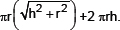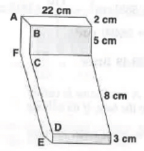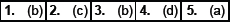# Surface Areas and Volumes-MCQ

Class X Math
MCQ for Surface Areas and Volumes
1.   If a solid, cone of base radius ‘r’ and height ‘h’ is placed over a solid cylinder having same base radius ‘r’ and height – ‘h’ as that of the cone, then the curved surface area of the shape isIs it true?
(a) No
(b) Yes
(c) May be
(d) Cannot be determined
2.   A cylinder and a cone are of the same base radius and same height. Find the ratio of the volumes of the cylinder of that of the cone.
(a) 1 : 3
(b) 1 : 2
(c) 3 : 1
(d) 2 : 1
3.   In the figure, the shape of a solid copper piece (made of two pieces) with dimensions as shown. The face ABCDEFA has uniform cross section. Assume that the angles at A, B, C, D, E and F are right angles. Calculate the volume of the piece.(a) 840 cm
(b) 880 cm3
(c) 876 cm3
(d) 890 cm3
4.   A toy is in the form of a cone mounted on a hemisphere of common base radius 7 cm. The total height of the toy is 31 cm. Find the total surface area of the toy.
(a) 465
(b) 912
(c) 769
(d) 858
5.   What is the area of a semi–circle of radius 5 cm?
(a) 78.57 cm
(b) 71.42 cm
(c) 63.18 cm
(d) 79.86 cm